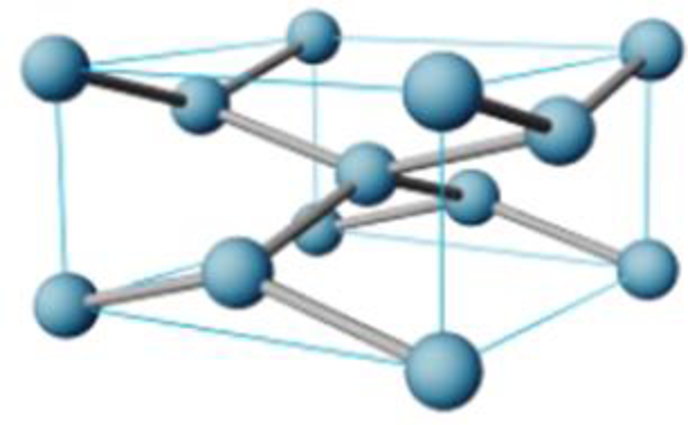Chapter 12.6, Problem 3.2ACP

Chapter
Section
Textbook Problem

Using the dimensions of the unit cell (Figure 2) and the molar mass of tin, calculate the density of white tin (in g/cm3).Figure 2 A tetragonal unit cell of white tin (β-tin). The tetragonal unit cod has 90° angles at each corner. Two of the three sides are equal in length (583 pm) while the third side is shorter (318 pm) (Note that the lines connecting atoms are not bonds They are meant only to show the spatial relationship of the atoms.)

Interpretation Introduction

Interpretation:

The density of the white tin has to be identified.

Concept introduction:

The density of the unit cell is calculated as follows,

Massvolume = density

Mass = number of moles × molar massNumber of moles = Number of atoms per unit cellAvogadro number

Explanation

Cube with a two side of white tin is 583 pm, and one side with 318 pm. The conversion of pm to cm is 5.83 × 10-8cm and 3.18 × 10-8cm

V=(a)3Cube with a two side of white tin is583 pm, and one side 318pmV=(5.83×108cm)2(3.18×10-8cm)V=1.081×10-22cm

Molar mass of tin is 118.7 gVolume of the unit cell  =1.081×10-22cm3

Mass = number of moles × molar massNumber of moles = Number of atoms per unit cellAvogadro number Number of moles = 46

Still sussing out bartleby?

Check out a sample textbook solution.

See a sample solution

The Solution to Your Study Problems

Bartleby provides explanations to thousands of textbook problems written by our experts, many with advanced degrees!

Get Started

A salt does not release ________ in water. a. ions b. H+

Biology: The Unity and Diversity of Life (MindTap Course List)

What impact has recombinant DNA technology had on genetics and society?

Human Heredity: Principles and Issues (MindTap Course List)

Which of these classifications likely contains the most biomass?

Oceanography: An Invitation To Marine Science, Loose-leaf Versin